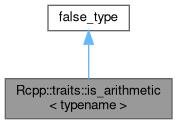Rcpp Version 1.0.7
Rcpp::traits::is_arithmetic< typename > Struct Template Reference

`#include <is_arithmetic.h>`

Inheritance diagram for Rcpp::traits::is_arithmetic< typename >:[legend]
Collaboration diagram for Rcpp::traits::is_arithmetic< typename >:[legend]Public Types inherited from Rcpp::traits::integral_constant< _T, _V >
typedef _T value_type

typedef integral_constant< _T, _V > typeStatic Public Attributes inherited from Rcpp::traits::integral_constant< _T, _V >
static const _T value = _V

## Detailed Description

### template<typename> struct Rcpp::traits::is_arithmetic< typename >

Definition at line 30 of file is_arithmetic.h.

The documentation for this struct was generated from the following file: